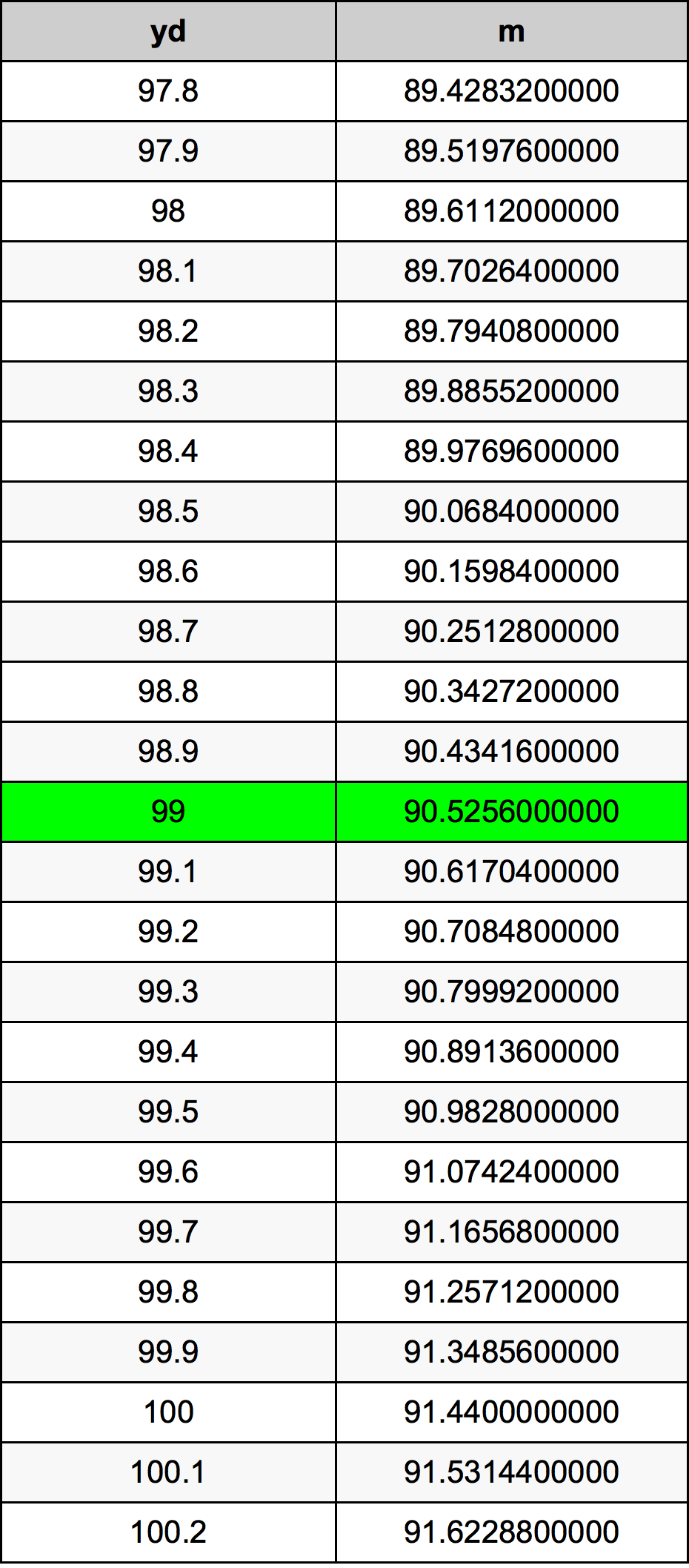Yards To Meters

# 99 yd to m99 Yards to Meters

yd
=
m

## How to convert 99 yards to meters?

 99 yd * 0.9144 m = 90.5256 m 1 yd
A common question is How many yard in 99 meter? And the answer is 108.267716535 yd in 99 m. Likewise the question how many meter in 99 yard has the answer of 90.5256 m in 99 yd.

## How much are 99 yards in meters?

99 yards equal 90.5256 meters (99yd = 90.5256m). Converting 99 yd to m is easy. Simply use our calculator above, or apply the formula to change the length 99 yd to m.

## Convert 99 yd to common lengths

UnitLength
Nanometer90525600000.0 nm
Micrometer90525600.0 µm
Millimeter90525.6 mm
Centimeter9052.56 cm
Inch3564.0 in
Foot297.0 ft
Yard99.0 yd
Meter90.5256 m
Kilometer0.0905256 km
Mile0.05625 mi
Nautical mile0.0488799136 nmi

## What is 99 yards in m?

To convert 99 yd to m multiply the length in yards by 0.9144. The 99 yd in m formula is [m] = 99 * 0.9144. Thus, for 99 yards in meter we get 90.5256 m.

## 99 Yard Conversion Table## Alternative spelling

99 yd to Meters, 99 yd in Meters, 99 Yards to Meter, 99 Yards in Meter, 99 yd to Meter, 99 yd in Meter, 99 Yards to Meters, 99 Yards in Meters, 99 Yard to m, 99 Yard in m, 99 yd to m, 99 yd in m, 99 Yard to Meters, 99 Yard in Meters